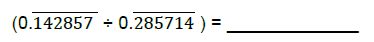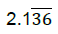Welcome to your Level 04 Practice VM Sr

Name
1.
Convert 3/25 into Decimals

2.
Subtract 5b-5a from 8b

3.
If the side of a cubiod is ( x+1), ( 2x+4) and (x+3). Find its volume

4.

x^2 +8x+16

5.
The length of a rectangle is 5 inches more than twice a number. The width is 4 inches less than the same number. If the area of the rectangle is 15, find the number?

6.
The length of a rectangle is 5 inches more than twice a number. The width is 4 inches less than the same number. If the area of the rectangle is 15, find the number:

7.
A match attracts 88 fans and draws in $553 in revenue from ticket sales. A child's ticket costs$4 and an adult's ticket is \$7. How many of each type of ticket were sold?"

8.
Find the value of x and y in the below equations by Vedic method:

3x - 7y = -10
y-2x = 3

9.
Find the value of x and y in the below equations by general method:method:

x + y/2 = 4
x/3 + 2y = 5

10.
Find what was day on 19th April 1943.

11.
Solve the following12.
Convert into fraction:13.
Find the cube root of 7444122

14.
Write the following phrases using literals, numbers and basic mathematical operations:

Age of Ravi after three years from now